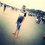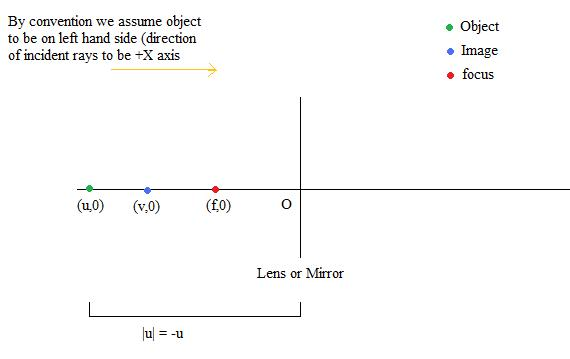# Sign Convention in Optics

In optics, sign, while deriving its formulas, for example the mirror formula $\rightarrow \dfrac{1}{f} = \dfrac{1}{v} + \dfrac{1}{u}$ , we use sign convention and while solving numericals, we again use sign convention.

So, why do we apply sign convention twice?Note by Akhil Bansal
5 years, 9 months ago

This discussion board is a place to discuss our Daily Challenges and the math and science related to those challenges. Explanations are more than just a solution — they should explain the steps and thinking strategies that you used to obtain the solution. Comments should further the discussion of math and science.

When posting on Brilliant:

• Use the emojis to react to an explanation, whether you're congratulating a job well done , or just really confused .
• Ask specific questions about the challenge or the steps in somebody's explanation. Well-posed questions can add a lot to the discussion, but posting "I don't understand!" doesn't help anyone.
• Try to contribute something new to the discussion, whether it is an extension, generalization or other idea related to the challenge.

MarkdownAppears as
*italics* or _italics_ italics
**bold** or __bold__ bold
- bulleted- list
• bulleted
• list
1. numbered2. list
1. numbered
2. list
Note: you must add a full line of space before and after lists for them to show up correctly
paragraph 1paragraph 2

paragraph 1

paragraph 2

[example link](https://brilliant.org)example link
> This is a quote
This is a quote
    # I indented these lines
# 4 spaces, and now they show
# up as a code block.

print "hello world"
# I indented these lines
# 4 spaces, and now they show
# up as a code block.

print "hello world"
MathAppears as
Remember to wrap math in $$ ... $$ or $ ... $ to ensure proper formatting.
2 \times 3 $2 \times 3$
2^{34} $2^{34}$
a_{i-1} $a_{i-1}$
\frac{2}{3} $\frac{2}{3}$
\sqrt{2} $\sqrt{2}$
\sum_{i=1}^3 $\sum_{i=1}^3$
\sin \theta $\sin \theta$
\boxed{123} $\boxed{123}$

Sort by:

- 5 years, 9 months ago

Hi @Akhil Bansal, I am having trouble understanding your question. Can you rephrase or include some more details?

Staff - 5 years, 9 months ago

Here is the derivation of mirror formula.. During deriving mirror formula, we use sign convention.

Now suppose we have to solve a numerical using mirror formula.... Example : An object is kept at distance 2m in front of a concave length of focal length 1 m. What is the image position?
Solution :Applying sign convention: u (object distance) = -2 m , f (focal length) = -1 m. , v(image distance) = ?
Using formula $\dfrac{1}{f} = \dfrac{1}{v} + \dfrac{1}{u}$.
$\dfrac{1}{-1} = \dfrac{1}{v} + \dfrac{1}{-2}$.
$v = -2m$.

Now, we have used the sign convention twice.First during deriving the formula, and second time while using it in numerical. Why so?

- 5 years, 9 months ago

Is your thinking like "if we apply the convention once in the derivation, and then once more in the application, the sign convention will be wiped out (two negatives make a positive)"?

Staff - 5 years, 8 months ago

Yup

- 5 years, 8 months ago

Hi @Akhil Bansal, I think there is a simpler way to obtain the result that avoids this confusing sign convention business. If we call $d_o$ the object distance, $d_i$ the image distance, and $f$ the focal length, then using similar triangles, it is easy to show

$\frac{f}{d_i-f} = \frac{d_o-f}{f}$

Note that each of these variables are physical horizontal distances measured in a coordinate system whose origin is at the surface of the mirror (positive in front of the mirror, negative behind). There is no sign convention, just a straightforward use of a coordinate.

That equation can be rearranged to give you the usual result $f^{-1} = d_o^{-1} + d_i^{-1}$.

It is clear that a mirror that curves toward the object will produce an image in on the same side of the mirror as the object (i.e. the focal point is in front of the mirror), and a mirror that curves away from the object will produce an image on the other side of the mirror (focal point is behind the mirror).

There is no need for a sign convention, just figure out where the focal point is, and use the coordinate system to measure the distance to it.

Staff - 5 years, 8 months ago

Thanks sir..

- 5 years, 8 months ago

Staff - 5 years, 8 months ago

Yes, i am satisfied..

- 5 years, 8 months ago

Hey See my comment @Akhil Bansal

- 5 years, 8 months ago

- 4 years, 8 months ago

Thak you soo much.. I understood it clearly.

- 4 years ago

Personally aometimes I do feel these confusing

- 5 years, 9 months ago

. The first time when sign convention is applied to derive Lens Formula, it is to make sure the formula is as general as possible. Meaning you may start deriving the formula for Convex lens but since you have ensured correct sign convention is used, it turns out the formula is valid for Concave lens too.In simple language you generalize the formula by doing that.As their are different formulas in different conditions in a mirror. That's what my logic says Please correct me if I am wrong

- 5 years, 9 months ago

Let's see if I can clear it @Akhil Bansal

NOTE

In the mirror and lens formula that we use, v,u and f is are not distances (of image, object and focus resp.)

They are coordinates

Hence, in the derivation when you take distances, you have to take modulus of the coordinates

and in the formula, put coordinates- 5 years, 8 months ago

@Akul Agrawal but you didnt clear the doubt that even if we take the modulus, we still apply -ve in derivation of formula. Then why should we apply -ve sign again when doing questions.

- 5 years, 8 months ago

Lets try to understand the solution in deep. Actually,say, we are talking of u which is,say, negative.

Then in the derivation because we have to put only length which is always positive, we will have to put

-u. But NOTE we have taken u to be negative at each step of our derivation. So while applying the formula, obviously we will put u negative.

clear? @Satyajit Ghosh , @Akhil Bansal

- 5 years, 8 months ago

You are right, but your explaination is not according to my question. Please read the question again..

- 5 years, 8 months ago

I think I have answered your question. Please read it carefully. If I conclude in one line, we are not applying sign convention twice. We are applying it only once.

Remember derivation of formula is purely mathematical without any sign convention

You may verify by taking different u and v (+ve and -ve), that the formula remains the same.

then we find the union of all the situations and say the formula to be true for all cases.

eg. if u=-1, we put u=-1 in formula as we took u to be -ve in derivation

and if u=1, we put u=1 in formula as we took u to be +ve in derivation

Note u and v are coordinates and not distances

- 5 years, 8 months ago

You are confusing me. $> Remember derivation of formula is purely mathematical without any sign convention.$ Well if sign conventions are not applied then we cannot even derive the formula. link given by @Akhil Bansal

- 5 years, 8 months ago

ACTUALLY WHILE DERIVING WE ARE NOT APPLYING SIGN CONVENTION. WE ARE APPLYING IT JUST WHEN WE CHOOSE SIGN OF U,V OR F I.E. COORDINATES OF PT OBJECT, PT IMAGE AND FOCUS RESP.

- 5 years, 8 months ago

Thats what is the doubt. If $> WE ARE APPLYING IT JUST WHEN WE CHOOSE SIGN OF U , V OR F I.E. COORDINATES OF PT OBJECT, PT IMAGE AND FOCUS RESP.$ Then why apply the signs again when doing questions?

- 5 years, 8 months ago

we are putting values of the same sign we chose before deriving. What's wrong with that?

- 5 years, 8 months ago

To be very precise, just remember that u,v and f are coordinates and forget everything about sign convention. This is the best solution i can tell @Akhil Bansal and @Satyajit Ghosh

because sign convention only says that we must take +ve x axis in direction of incident rays.

- 5 years, 8 months ago